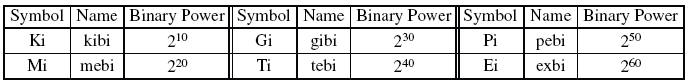Binary Multiples

The International Electrotechnical Commission has assigned the following prefixes to represent exponential binary multiples. This avoids confusion with standard SI decimal prefixes when representing powers of 2, as in bits and bytes.Example 1:2 Ki = 2 × 210 = 2 × 1,024 = 2,048. This does not equal 2 K = 2 × 103 = 2,000.

Example 2:1 mebibyte = 1 × 220 = 1,048,576 bytes. Again this does not equal 1 megabyte = 1 × 106 = 1,000,000 bytes, a value that is often confused with 1,048,576 bytes.

Recherche personnalisée